Digital Logic Gates Part-V Jan-4-2006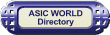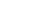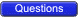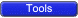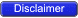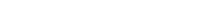Diode Logic In DL (diode logic), all the logic are implemented using diodes and resistors. One basic thing about the diode, is that diode needs to be forward biased to make it conduct. Below is the example of few RTL logic circuits.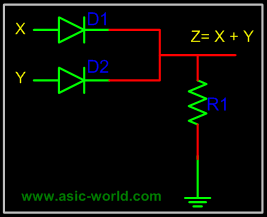When there is no input is connected or driven, output Z is low, due resistor R1. When high is applied to either X or Y, or Both X and Y are driven high, corresponding diode get forward biased and thus making diodes to conduct. When anyone one the diode conduct, out Z goes high.Points to Ponder Diode Logic suffers from voltage degradation from one stage to the next. Diode Logic only permits the OR and AND functions. Diode Logic is used extensively but not in integrated circuits.Resistor Transistor Logic In RTL (resistor transistor logic), all the logic are implemented using resistors and transistors. One basic thing about the transistor (NPN), is that HIGH at input causes output to be LOW (i.e. like a inverter). Below is the example of few RTL logic circuits.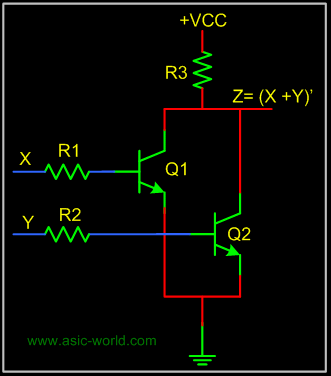A basic circuit of an RTL NOR gate consists of two transistors Q1 and Q2, connected as shown in figure above. When either of input X or Y is driven HIGH, corresponding transistor is goes to saturation and output Z is pulled to LOW.Diode Transistor LogicIn DTL (Diode transistor logic), all the logic are implemented using diodes, and transistors. Basic circuit in the DTL logic family is as shown in figure below. Each of the input is associated with one diode. The diodes and the 4.7K resistor form an AND gate. If input X, Y, Z is low, the corresponding diode conducts current, thorough the 4.7K resistor. Thus there is no current through the diodes connected in series to transistor base . Hence the transistor does not conduct, thus remains in cut-off, and output out is High.If all the inputs X, Y , Z are driven high, the series diodes conduct and thus driving transistor into saturation. Thus output out is Low.Transistor Transistor Logic Transistor Transistor logic or just TTL, logic gates are built around only transistors. TTL was developed in 1965. Through the years basic TTL has been improved to meet the performance requirements. There are many versions or families of TTLStandard TTL. High Speed TTL Low Power TTL. Schhottky TTL.Here we will discuss only basic TTL as of now, may be in future, I will add more details about other versions of TTL. As such all the families of TTL have three configuration for outputs.Totem - Pole output. Open Collector Output. Tristate Output.Before we discuss the output stage lets looks at the input stage, which is used almost with all the versions of TTL. This consists of input transistor and phase splitter transistor. Input stage consists of multi emitter transistor as shown in figure below. When any of the input is driven low, i.e. emitter base junction is forward biased and input transistor conducts. This in turn drives the phase splitter transistor into cut-off.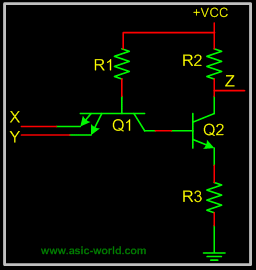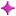Totem - Pole Output Below is the circuit of a totem-pole NAND gate, which has got three stages.Input Stage Phase Splitter Stage Output StageInput stage and Phase splitter stage has already been discussed. Output stage is called Totem-Pole because, the transistor Q3 sites upon Q4.Q2 provides complementary voltages for the output transistors Q3 and Q4, which stacked one above the other in such a way that while one of these conducts, the other is cut-off.Q4 is called pull-down transistor, as it pulls the output voltage down, when it saturates and other is in cut-off (i.e. Q3 is in cut-off). Q3 is called pull-up transistor, as it pulls the output voltage up, when it saturates and other is in cut-off (i.e. Q4 is in cut-off).Diodes in input are protection diodes, which conduct when there is large negative voltage at input. Thus shorting the negative voltage to ground.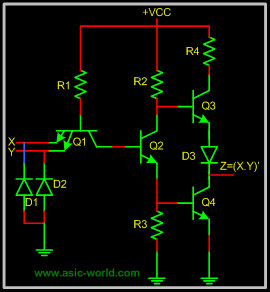Tristate Output. Normally when we have to implement shared bus system inside a ASIC or external to the chip, we have two options, either to use MUX/DEMUX based system or, use tri-state base bus system.In later, when a logic is not driving it output, it does not drive LOW and also does not drive HIGH. Which means the output of the logic is floating. Well one may ask, why not just use open collector for shared bus systems. The problem is open collector is not so good to implement wire-AND's.Below circuit is tri-state NAND gate, when Enable En is HIGH, below circuits works like any other NAND gate. But when Enable En is driven LOW. Q1 Conducts, and diode connecting emitter of Q1 and collector or Q2 conducts. Thus driving Q3 into cut-off. Since Q2 is not conducting, Q4 is also at cut-off. When both the pull-up and pull-down transistors are not conducting, out Z is in high-impedance state.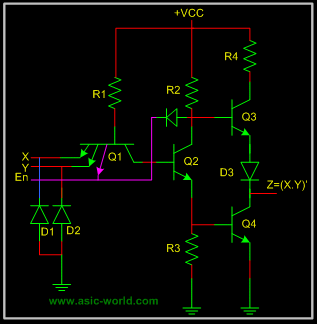Note : I will try to add more details when I find time.Copyright © 1998-2006 Deepak Kumar Tala - All rights reserved Do you have any Comment? mail me at: deepak@asic-world.com